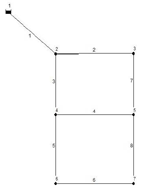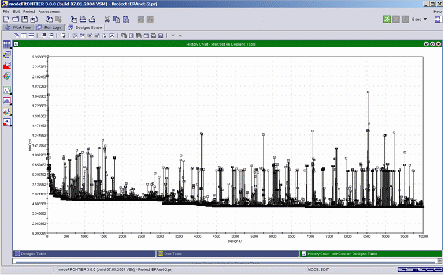# Optimization of a Two Loop Pipe Network

Author(s):

The two loop pipe network, shown in Figure 1, has been used as a test problem for optimization algorithms by a number of researchers (see, for example,  and ). Here we describe how the problem was solved using genetic algorithms available in the commercial optimization software, modeFRONTIER, coupled with Epanet 2, a hydraulics program developed by the U.S. Environmental Protection Agency (EPA). Epanet 2 is downloadable, free of charge, from the EPA’s website (http://www.epa.gov).SOBOL was chosen as the DOE scheduler to create the initial 10 designs, used to populate the design space, and, due to the fact that we have discrete variables, MOGA (the Multiobjective Genetic Algorithm in modeFRONTIER) was chosen as the optimization algorithm. A total of 1000 generations were run (10,000 calculations), each of which took of the order of 1 second on a 2.4 GHz P4 machine.

Fig. 1 Two loop pipe networks

The history chart for the total cost is shown in Figure 2. The minimum value obtained for the total cost, reached after around 6000 designs, was 419,000 – exactly the same as the minimum value obtained in .Fig. 2 History Chart for Total Cost